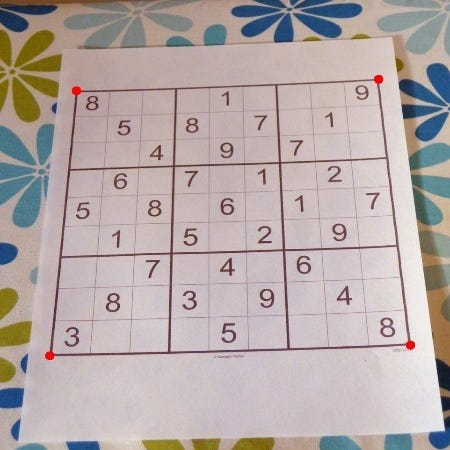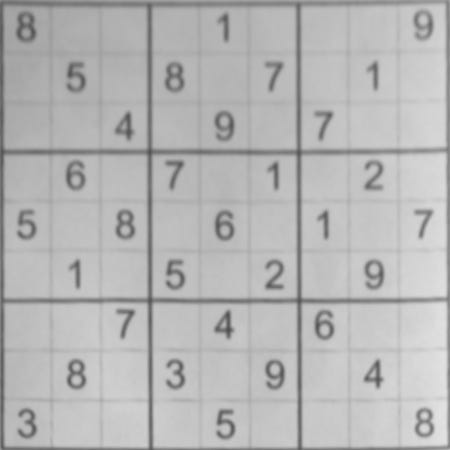# Sudoku Solver using OpenCV and DL — Part 1

Source: Deep Learning on Medium

If you’re impatient, scroll to the bottom of the post for the Github Repos

I, like many others, enjoy solving new puzzles and questions. During my school days, each morning I used to do The Times of India’s sudoku. Everyone knows how to solve sudoku but have you ever wondered that you can get to the solution without even scratching your head once. You just have to click the picture of the sudoku and it should calculate the solution for you.

Peter Norvig in his Solving Every Sudoku Puzzle gives a beautiful summary of the game’s rule in just one sentence.

A puzzle is solved if the squares in each unit are filled with a permutation of the digits 1 to 9.

#### The rules of the game:

It’s all about putting digits between 1 and 9 into a square, 9×9 grid, subdivided into 9 boxes. But The value of a cell cannot be repeated amongst any of its peers.

Now that you know the rules, let’s do the heavy lifting so that you don’t have to spend minutes or maybe hours solving the sudoku. Again what we have to do? Take this image and somehow extract the sudoku from this image and calculate the solution.

We will be dividing the whole process into 3 steps.

A: Extract the sudoku from the image

B: Extract each number present in the image

C: Compute the solution of the sudoku using algorithm

### A: Extract the sudoku from the image

We need to do a bit of image processing before proceeding further.

#### 1: Pre Processing the image

First, we apply Gaussian blur with a kernel size (height, width) of 9 to the image. Note that kernel sizes must be positive and odd and the kernel must be square. Then we use adaptive threshold using 11 nearest neighbour pixels.

`proc = cv2.GaussianBlur(img.copy(), (9, 9), 0)proc = cv2.adaptiveThreshold(proc, 255, cv2.ADAPTIVE_THRESH_GAUSSIAN_C, cv2.THRESH_BINARY, 11, 2)`

To make gridlines have non-zero pixel values, we will invert the colours. Also, we will dilate the image to increase the size of gridline.

`proc = cv2.bitwise_not(proc, proc) kernel = np.array([[0., 1., 0.], [1., 1., 1.], [0., 1., 0.]],np.uint8)proc = cv2.dilate(proc, kernel)`

#### 2: Find the corners of the largest polygon

Next step is to find the 4 extreme corners of the largest contour in the image. So we will find all the contours, sort by area in descending order and pick the one with the largest area.

`_, contours, h = cv2.findContours(img.copy(), cv2.RETR_EXTERNAL, cv2.CHAIN_APPROX_SIMPLE)contours = sorted(contours, key=cv2.contourArea, reverse=True)polygon = contours`

Use of `operator.itemgetter` with `max` and `min` allows us to get the index of the point. Each point is an array of 1 coordinate, hence the  getter, then  or  used to get x and y respectively.

Bottom-right point has the largest (x + y) value. Top-left has point smallest (x + y) value. The bottom-left point has the smallest (x — y) value. The top-right point has the largest (x — y) value.

`bottom_right, _ = max(enumerate([pt + pt for pt in polygon]), key=operator.itemgetter(1))top_left, _ = min(enumerate([pt + pt for pt in polygon]), key=operator.itemgetter(1))bottom_left, _ = min(enumerate([pt - pt for pt in polygon]), key=operator.itemgetter(1))top_right, _ = max(enumerate([pt - pt for pt in polygon]), key=operator.itemgetter(1))`

Now that we have all the 4 coordinates. We will return an array of all the 4 points using the indices. Each point is in its own array of one coordinate.

`[polygon[top_left], polygon[top_right], polygon[bottom_right], polygon[bottom_left]]`4 corners of the largest polygon

#### 3: Crop and Warp Image

Now that we have all the 4 coordinates of sudoku, we will crop and warp a rectangular section from an image into a square of similar size. The rectangle described by the top left, top right, bottom right and bottom left points.

Note: Explicitly set the data type to float32 or `getPerspectiveTransform` will throw an error.

`top_left, top_right, bottom_right, bottom_left = crop_rect, crop_rect, crop_rect, crop_rectsrc = np.array([top_left, top_right, bottom_right, bottom_left], dtype='float32') side = max([ distance_between(bottom_right, top_right),  distance_between(top_left, bottom_left), distance_between(bottom_right, bottom_left),  distance_between(top_left, top_right) ])`

We will describe a square with side of the calculated length, this is the new perspective we want to warp to. Next thing is to get the transformation matrix for skewing the image to fit a square by comparing the 4 before and after points. In the end, we will perform the transformation on the original image.

`dst = np.array([[0, 0], [side - 1, 0], [side - 1, side - 1], [0, side - 1]], dtype='float32')m = cv2.getPerspectiveTransform(src, dst)cv2.warpPerspective(img, m, (int(side), int(side)))`Sudoku image after crop and warp perspective transform

#### 4: Infer grid from the square image

Infers 81 cell grid from a square image. We swap j and i here so the rectangles are stored in the list reading left-right instead of top-down.

`squares = [] side = img.shape[:1] side = side / 9for j in range(9): for i in range(9): p1 = (i * side, j * side) #Top left corner of a box  p2 = ((i + 1) * side, (j + 1) * side) #Bottom right corner  squares.append((p1, p2)) return squares`

#### 5: Get each digit

Next step is to extracts digits from their cells and builds an array.

`digits = []img = pre_process_image(img.copy(), skip_dilate=True)for square in squares: digits.append(extract_digit(img, square, size))`

extract_digit is a function which extracts a digit (if one exists) from a Sudoku square. It gets the digital box from the whole square, use the fill feature finding to get the largest feature in the middle of the box. We would expect to find a pixel belonging to the digit in the margin which is used to define an area in the middle. Next, we will scale and pad the digit so that it fits a square of the digit size we’re using for machine learning. Also, we have to ignore any small bounding boxes

`def extract_digit(img, rect, size): digit = cut_from_rect(img, rect)`
` h, w = digit.shape[:2] margin = int(np.mean([h, w]) / 2.5) _, bbox, seed = find_largest_feature(digit, [margin, margin], [w - margin, h - margin]) digit = cut_from_rect(digit, bbox)  w = bbox - bbox h = bbox - bbox if w > 0 and h > 0 and (w * h) > 100 and len(digit) > 0: return scale_and_centre(digit, size, 4) else: return np.zeros((size, size), np.uint8)`